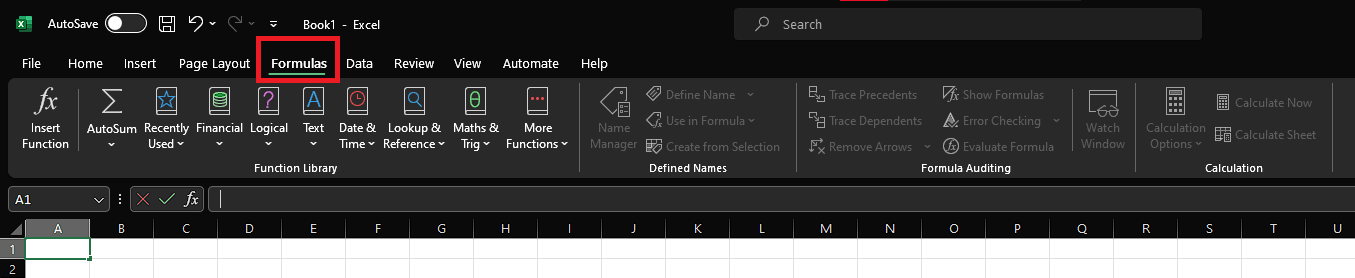Course Content

# Excel Beginner Course

Excel Beginner Course

##Different Types of Functions

Now that we have understood what functions and formulas are, we are going to understand some of the major categories for functions.

1. Mathematical Functions: These functions perform basic mathematical operations and are among the most commonly used in Excel. Examples: `SUM`, `AVERAGE`, `MIN`, `MAX`
2. Text Functions: Text functions manipulate and format text data in Excel.Examples: `CONCAT`, `LEN`, `LEFT`, `RIGHT`, `UPPER`
3. Date and Time Functions: These functions are used for date and time calculations and formatting. Examples: `TODAY`, `NOW`, `DATE`, `TIME`, `DATEDIF`
4. Logical Functions: Logical functions are used to evaluate conditions and return results based on those conditions.Examples: `IF`, `AND`, `OR`, `NOT`
5. Lookup and Reference Functions: These functions are used to search for specific values within a range or retrieve data from other cells.Examples: `VLOOKUP`, `INDEX`, `MATCH`;
6. Statistical Functions: Statistical functions help analyze data by providing various statistical measures. Examples: `COUNT`, `STDEV`, `RANK`
7. Financial Functions: These functions are useful for financial and economic calculations. Examples: `PV`, `FV`, `IRR`.

You can access all these functions (together with the official documentation) from the Formulas section:We will play around with some of the above formulas, but for now, we reached the end of this chapter.

Let’s answer to this quiz in order to move forward to the next one:

1. Which type of functions is used for financial and economic calculations in Excel?
2. Try it in your own workbook. Try to use the CONCAT function to concatenate two cells containing 2 numbers. Does Excel allow it?